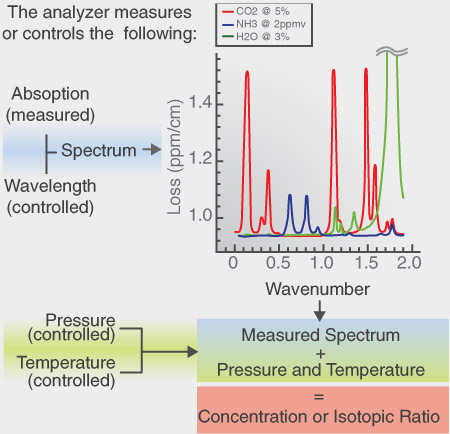## i) Absolute wavelength monitoring

Pushing CRDS to the parts per trillion (ppt) level requires very precise scanning and fitting of the target absorption line. The wavelength of the available near-IR laser diodes is directly tuned by changing its drive current and temperature. However, the exact relationship between current and wavelength varies from laser to laser. Moreover, because this relationship can change over time, the wavelength cannot be known from the drive current alone. Additionally, it is necessary to know the absolute value of the laser wavelength to a precision that is a few orders of magnitude narrower than the spectral linewidth. Commercial wavemeters, however, do not offer this precision. This is the main reason that older, competitive cavity-based instruments try to lock to a single wavelength rather than scan across the entire absorption line.

At Picarro we solved this problem by developing and patenting our own wavelength monitor. It can measure absolute laser wavelength to a precision more than 1000 times narrower than the observed Doppler-broadened linewidth for small gas phase molecules. We then use this in a novel way - specifically, we lock the laser to the wavemeter, which we then actively tune to known wavelengths. The result is higher spectral precision than in any commercial spectrometer - laser-based or otherwise. This spectral precision is the key to the ultra-precise fitting of lineshapes and line heights necessary to reach parts per trillion concentration sensitivity.

## ii) Precise pressure and temperature control

The relationship between the observed line intensity and the true concentration depends on both the pressure and temperature of the sample gas. Accurate absorption measurements at precisely known wavelengths account for little unless the temperature and pressure of the CRDS measurement cavity are known. To completely minimize instrument measurement drift, however, these parameters must not only be known, but they also must be actively stabilized to constant values.

In Picarro CRDS gas analyzers, the sample cavity is surrounded by layers of thermally insulating material to provide a high degree of passive thermal stability. The cavity is further actively stabilized by means of a solid-state heating system locked to the output of a thermal sensor. This enables the cavity to be permanently locked to better than 20 mK.

The sample pressure in the cavity is sensed using a high-linearity pressure transducer. The system computer uses this pressure data in a feedback loop to control the proportional valves which adjust the inlet and outlet gas flow of the cavity. In this way, the pressure in Picarro analyzers is actively stabilized to better than 1 part in 2000.

The bottom line: by measuring and controlling laser wavelength, sample pressure and temperature, Picarro instruments can reach ppbv to pptv sensitivity. And just as important, the long term (30-day) measurement drift is typically at the ppbv level. This enables a CRDS instrument to be operated for several months (or in some cases over a year) before any re-calibration is required.Schematic showing how the Picarro analyzer achieves high sensitivity and stability. The wavelength, temperature and pressure are precisely controlled, and the time-based ring down measurement accurately determines the concentration. The sample spectrum shows how individual absorption peaks can be selected so that they do not overlap with peaks from interfering gas species. In cases where interferences are unavoidable, interfering peaks can themselves be measured and their contributions to the measurement of interest deconvolved and eliminated form the result.

## iii) Key CRDS Formulas

The following notes briefly outline the calculations used to convert ring down times into absorption intensity. This is done automatically in Picarro CRDS analyzers and is included here only for completeness.

The light signal at the photodetector is given by

I(t, λ) = I0 e-t/τ( λ)

Where I0 is the transmitted light intensity at the time the laser is switched off and τ(λ) is the ring down time constant. For a given wavelength, λ, the decay rate,

R (λ) = 1/τ(λ)

is proportional to the optical losses inside the cavity and equal to the empty-cavity decay rate plus a factor dependent on the sample absorption:

R (λ,C) = 1/(λ) = R (λ,O) + cε(λ)C

where R(λ) = 1/τo(λ) is the empty-cavity decay rate. The effective path length of the measurement is given by

Leff = cτo(λ)

where c is the speed of light. For typical mirrors having a reflectivity of 99.995% and scattering losses of less than 0.0005 percent, Leff can be over 10 km. For a cell length of 25cm, this is a pathlength enhancement factor of over 20,000.

The sample absorption can be written as:

α(λ) = ε(λ)C

Where ε is the extinction coefficient and C is the concentration. This can be found by taking the difference between the decay rates of an empty cavity (C = 0) and a cavity containing a sample, i.e.:

α(λ) = 1/c[R(λ,c) - R(λ,0)]

If the absorption cross section and lineshape parameters of the sample are known, then the concentration of the sample can be readily computed. For a more detailed mathematical treatment of cavity ring down spectroscopy, see K.W. Busch and M.A. Busch (1997). "Cavity ring-down spectroscopy: An ultratrace absorption measurement technique." ACS Symposium Series 720, Oxford.